# 【算法面试】leetcode最常见的150道前端面试题 --- 中等题2（共80题）

## 378. 有序矩阵中第 K 小的元素(中等难度)

``````输入：matrix = [[1,5,9],[10,11,13],[12,13,15]], k = 8

``````

``````输入：matrix = [[-5]], k = 1

``````

• 它是一颗完全的二叉树，表示树的每一层都有左侧和右侧子节点（除了最后一层的叶子节点），并且最后一层的叶节点尽可能都是左侧子节点
• 二叉堆不是最小堆就是最大堆。最小堆允许你快速导出树的最小值，最大堆允许你快速导出树的最大值。所有的节点都大于等于最大堆或小于等于最小堆的每个它的子节点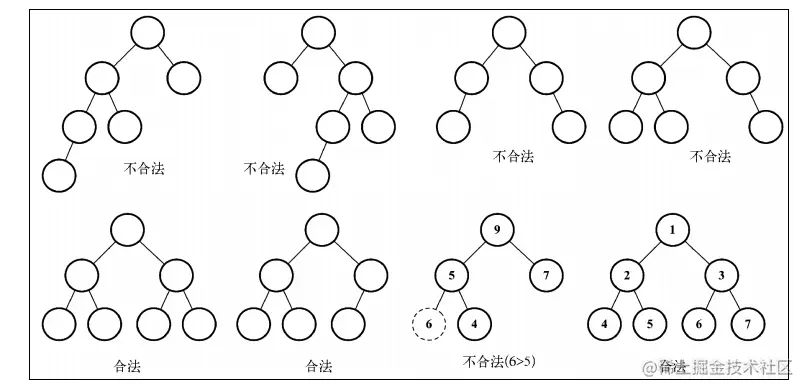我们用数组来模拟这样一个二叉堆。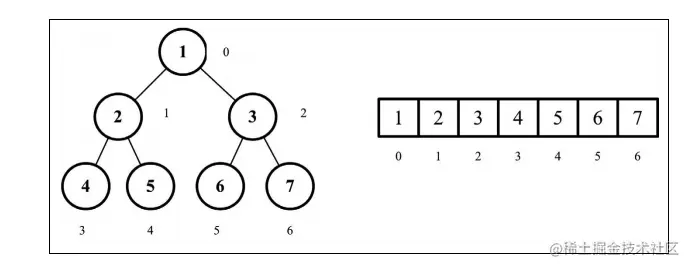• 它的左侧子节点的位置是 2 * index + 1
• 它的右侧子节点的位置是 2 * index + 2
• 它的父节点的位置是 (index-1) / 2 如果位置可用

``````const COMPARE_NUM = {
less: -1,
great: 1,
equal: 0,
}
function defaultCompreFn(i, j){
if(i - j === COMPARE_NUM.equal) return COMPARE_NUM.equal;
return i > j ? COMPARE_NUM.great : COMPARE_NUM.less;
}
``````

``````class MinHeap{
constructor(compareFn = defaultCompreFn){
this.heap = [];
this.compareFn = compareFn;
}
``````

``````  getLeftIndex(index){
return 2 * index + 1;
}

getRightIndex(index){
return 2 * index + 2;
}

getParentIndex(index){
if(index === 0) return;
return (index - 1) >> 1;
}
``````

``````  swap(i, j){
[this.heap[i], this.heap[j]] = [this.heap[j], this.heap[i]];
}
``````

``````  insert(value){
if(value !== null){
// 往数组末尾添加数据
this.heap.push(value);
// 添加的数据上移到堆合适的位置
this.siftUp(this.heap.length - 1);
return true;
}
return false;
}

siftUp(index){
let parentIndex = this.getParentIndex(index);
// index > 0 非常必要，因为index等于0就到了小顶堆的顶部了
while(index > 0 && this.compareFn(this.heap[index], this.heap[parentIndex]) === COMPARE_NUM.less) {
this.swap(index, parentIndex);
index = parentIndex;
parentIndex = this.getParentIndex(parentIndex);
}
}
``````

``````  extract(){
if(this.heap.length === 0) return;
if(this.heap.length === 1) return this.heap.shift();
const removedValue = this.heap;
this.heap = this.heap.pop();
// 下移从最后面放到第一位的元素
this.siftDown(0);
return removedValue;
}
``````

`````` siftDown(index) {
let tempIndex = index;
const leftIndex = this.getLeftIndex(index);
const rightIndex = this.getRightIndex(index);
if(leftIndex < this.heap.length && this.compareFn(this.heap[leftIndex], this.heap[tempIndex]) === COMPARE_NUM.less) {
tempIndex = leftIndex;
}
if(rightIndex < this.heap.length && this.compareFn(this.heap[rightIndex],this.heap[tempIndex]) === COMPARE_NUM.less) {
tempIndex = rightIndex;
}
if(tempIndex !== index){
this.swap(tempIndex, index);
this.siftDown(tempIndex);
}
}
}
``````

``````var kthSmallest = function(matrix, k) {
let result;
const length = matrix.length;
const heap = new MinHeap();
for(let i = 0; i < length; i++){
for(let j = 0; j < length; j++){
heap.insert(matrix[i][j])
}
}
while(k--){
result = heap.extract();
}

return result;
};
``````

## 删除链表的倒数第 N 个结点

• 首先设置快指针先走n步，到达从头数，链表第n个节点
• 慢指针从头开始走，跟快指针一起走，快指针到达链表尽头的时候，慢指针的下一个节点就是第n个节点
`````````javascript
var removeNthFromEnd = function(head, n) {
let slow = slowCopy = fast = new ListNode();
while(n--){
fast = fast.next;
}
while(fast.next){
slow = slow.next;
fast = fast.next;
}
slow.next = slow.next.next;
return slowCopy.next;
};
``````

## 36. 有效的数独

1. 数字 `1-9` 在每一行只能出现一次。
2. 数字 `1-9` 在每一列只能出现一次。
3. 数字 `1-9` 在每一个以粗实线分隔的 `3x3` 宫内只能出现一次。（请参考示例图）

• 一个有效的数独（部分已被填充）不一定是可解的。
• 只需要根据以上规则，验证已经填入的数字是否有效即可。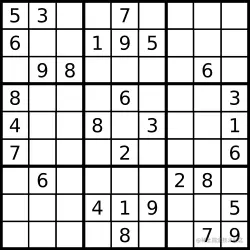``````输入：board =
[["5","3",".",".","7",".",".",".","."]
,["6",".",".","1","9","5",".",".","."]
,[".","9","8",".",".",".",".","6","."]
,["8",".",".",".","6",".",".",".","3"]
,["4",".",".","8",".","3",".",".","1"]
,["7",".",".",".","2",".",".",".","6"]
,[".","6",".",".",".",".","2","8","."]
,[".",".",".","4","1","9",".",".","5"]
,[".",".",".",".","8",".",".","7","9"]]

``````

``````输入：board =
[["8","3",".",".","7",".",".",".","."]
,["6",".",".","1","9","5",".",".","."]
,[".","9","8",".",".",".",".","6","."]
,["8",".",".",".","6",".",".",".","3"]
,["4",".",".","8",".","3",".",".","1"]
,["7",".",".",".","2",".",".",".","6"]
,[".","6",".",".",".",".","2","8","."]
,[".",".",".","4","1","9",".",".","5"]
,[".",".",".",".","8",".",".","7","9"]]

``````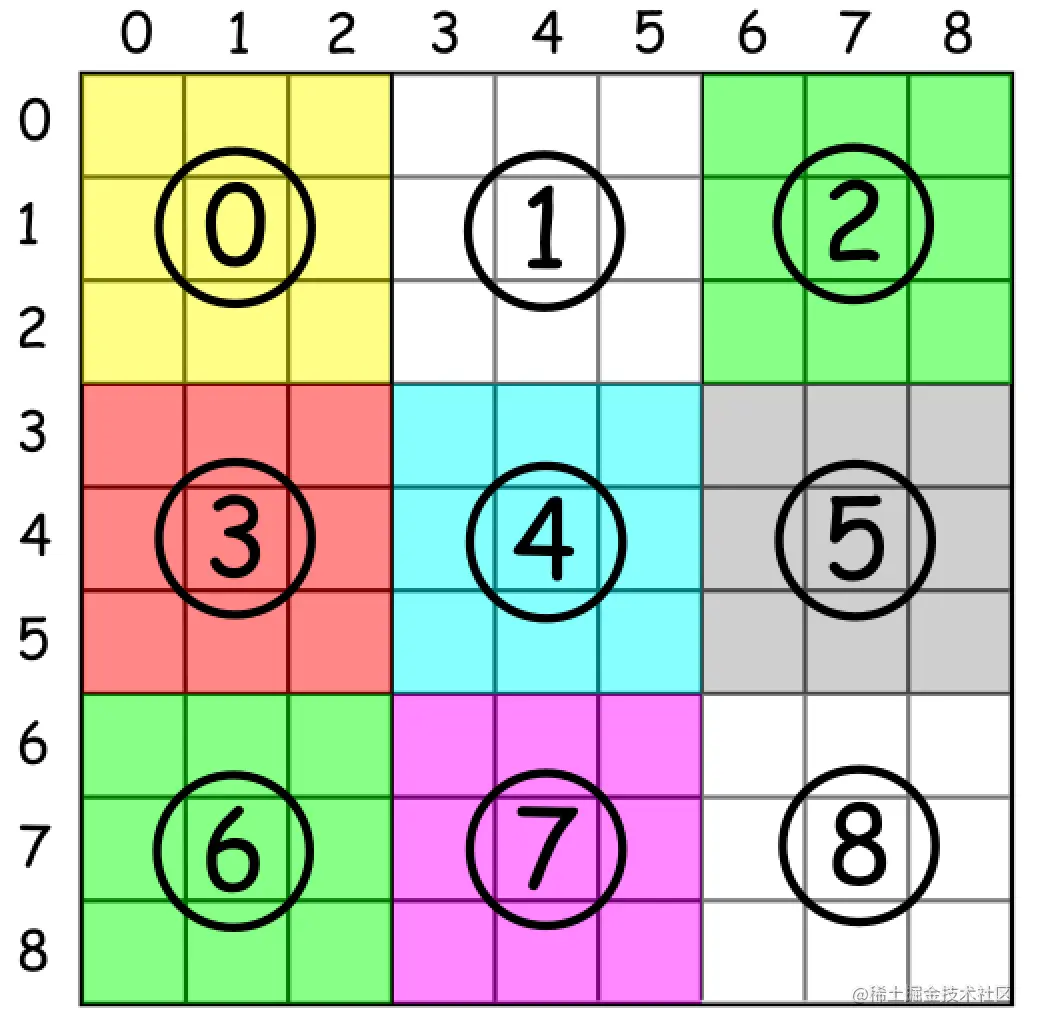3x3的验证关键就是横向坐标和竖直方向坐标都 %3 看是否 等于 0， 如果等于0，说明从这个位置开始，横向和纵向3x3就是我们要验证的区域。

``````var isExistInMap = function(
map, item) {
if(map[item] && item !== '.'){
return false;
} else {
map[item] = item;
return true;
}
};
var validateHV = function(nums) {
const validateMap = {};
return nums.every(num => isExistInMap(validateMap, num));
};

var validate3x3 = function(nums) {
const validateMap = {};
return nums.every(num => isExistInMap(validateMap, num));
};

var getVerticalNums = function(nums, row) {
const result = [];
for(let i = 0; i < nums.length; i++) result.push(nums[i][row])
return result;
};

var get3x3 = function(nums, row, col) {
const result = [];
for(let i = col; i < col + 3; i++) {
for(let j = row; j < row + 3; j++){
result.push(nums[i][j]);
}
}
return result;
};

var isValidSudoku = function(board) {
for(let i = 0; i < 9; i++) {
if(!validateHV(board[i])) return false;
if(!validateHV(getVerticalNums(board, i))) return false;
for(let j = 0; j < 9; j++) {
if(i % 3 === 0 && j % 3 === 0 && !validateHV(get3x3(board, i, j))) return false
}
}
return true;
};
``````

## 38. 外观数列

「外观数列」是一个整数序列，从数字 1 开始，序列中的每一项都是对前一项的描述。

• `countAndSay(1) = "1"`
• `countAndSay(n)` 是对 `countAndSay(n-1)` 的描述，然后转换成另一个数字字符串。

``````1.     1
2.     11
3.     21
4.     1211
5.     111221

``````

• 从第一个字符串开始，遍历字符串
• 如果前一个字符和后一个字符相同，就加在一起，直到后一个字符和前一个字符不一样
• 然后统计之前相同字符的个数，以此类推
``````var countAndSay = function(n) {
if(n === 1) return '1';
return generatorCount(countAndSay(n-1));

};
function generatorCount(n){
let initStr = n;
let result =''
for(let i = 0; i < n.length; i++){
if(n[i] === n[i+1]){
initStr += n[i+1]
}else{
result += initStr.length + initStr;
initStr = n[i+1];
}
}
return result;
}
``````

## 46. 全排列

``````输入： nums = [1,2,3]

``````

``````输入： nums = [0,1]

``````

``````输入： nums = 

``````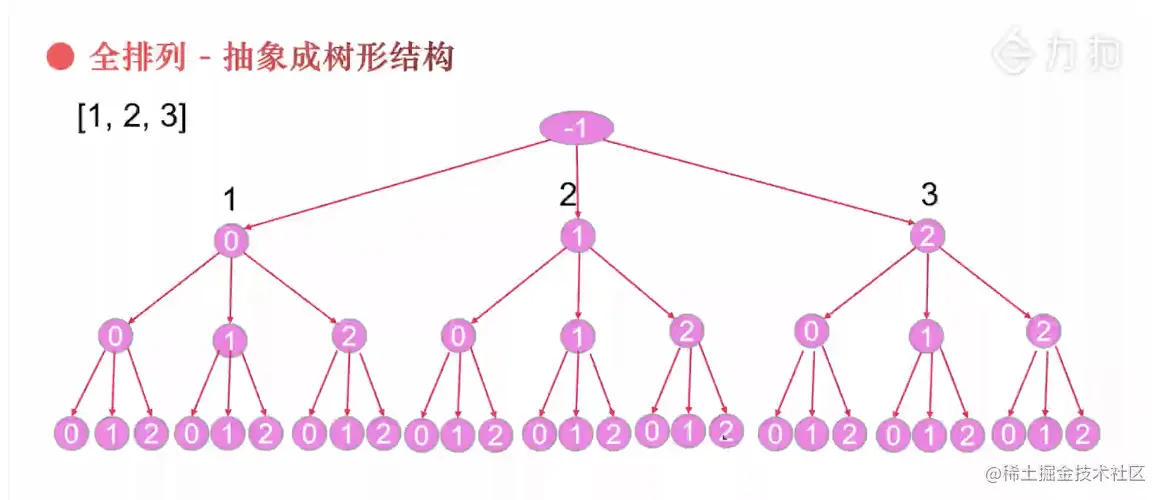• 回溯中参数为原始数组nums，以及当前数组arr
• 回溯的终止条件为，当前arr的个数已经等于原始数组nums
• 回溯的处理，循环遍历原始数组nums,当前值不存在arr中时，将该值放入arr中，递归调用
``````var permute = function(nums) {
const result = [];
function dfs(partialResult){
if(partialResult.length === nums.length){
result.push(partialResult);
return;
}
for(let i = 0, len = nums.length; i < len; i++){
if(partialResult.includes(nums[i])) { continue };
dfs(partialResult.concat(nums[i]));
}
}
dfs([]);
return result;
};
``````

## 旋转图像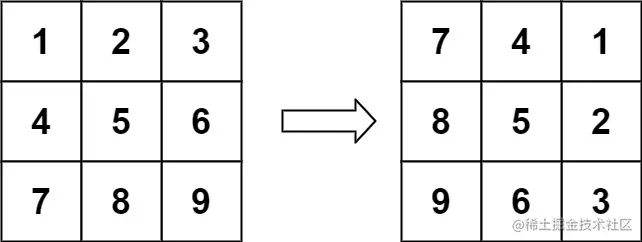``````输入： matrix = [[1,2,3],[4,5,6],[7,8,9]]

``````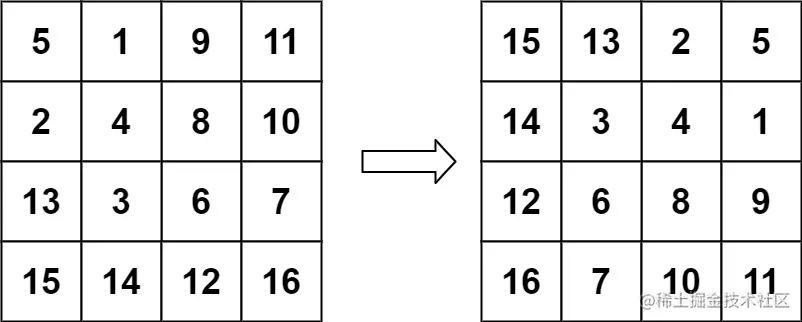``````输入：matrix = [[5,1,9,11],[2,4,8,10],[13,3,6,7],[15,14,12,16]]

``````

``````输入：matrix = []

``````

``````输入：matrix = [[1,2],[3,4]]

``````

``````1 2 3
4 5 6
7 8 9
``````

``````1 4 7
2 5 8
3 6 9
``````

``````7 4 1
8 5 2
9 6 3
``````

``````1 2 3
4 5 6
7 8 9
``````

``````1 4 7
2 5 8
3 6 9
``````

``````7 4 1
8 5 2
9 6 3
``````
``````var rotate = function(matrix) {
for(let i = 0; i < matrix.length; i++){
for(let j = i; j < matrix.length; j++){
[matrix[i][j], matrix[j][i]] = [matrix[j][i], matrix[i][j]]
}
}
return matrix.map(item => item.reverse());
};

``````

## 49. 字母异位词分组

``````输入: strs = ["eat", "tea", "tan", "ate", "nat", "bat"]

``````

``````输入: strs = [""]

``````

``````输入: strs = ["a"]

``````

``````var groupAnagrams = function(strs) {
const recordMap = {};
const result = [];
for(let str of strs){
const sortStr = str.split('').sort().join('');
if(recordMap[sortStr]){
recordMap[sortStr].push(str);
} else {
recordMap[sortStr] = [str];
}
}
for(let key in recordMap){
result.push(recordMap[key])
}
return result;
};
``````

## 2. 两数相加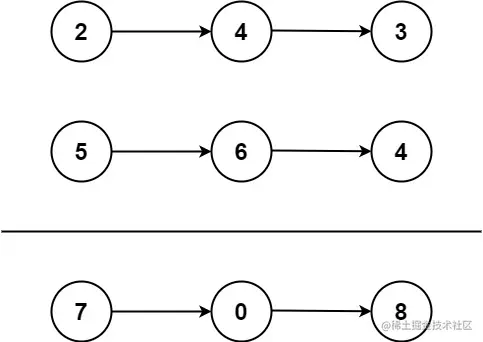``````输入：l1 = [2,4,3], l2 = [5,6,4]

``````

``````输入：l1 = , l2 = 

``````

``````输入：l1 = [9,9,9,9,9,9,9], l2 = [9,9,9,9]

``````

``````var addTwoNumbers = function(l1, l2) {
let carry= 0;
let pre = point =  new ListNode();
while(l1 || l2){
point.next = new ListNode();
point = point.next;
let sum = 0;
if(l1){
sum += l1.val;
l1 = l1.next;
}
if(l2){
sum += l2.val;
l2 = l2.next;
}
sum = sum + carry;
point.val = sum % 10;
carry = (sum / 10) | 0;
}
if(carry) point.next = new ListNode(carry);
return pre.next;
};
``````

## 3. 无重复字符的最长子串

``````输入: s = "abcabcbb"

``````

``````输入: s = "bbbbb"

``````

``````输入: s = "pwwkew"

请注意，你的答案必须是 子串 的长度，"pwke" 是一个子序列，不是子串。
``````

``````  ┌─┐
│a│b c a b c b b   # max = 0 , arr.length =1   取最大得：  max = 1
└─┘

┌───┐
│a b│c a b c b b   # max = 1 , arr.length =2   取最大得：  max = 2
└───┘

┌─────┐
│a b c│a b c b b   # max = 2 , arr.length =3   取最大得：  max = 3
└─────┘

┌─────┐
a│b c a│b c b b   # max = 3 , arr.length =1   取最大得：  max = 3
└─────┘

┌─────┐
a b│c a b│c b b   # max = 3 , arr.length =1   取最大得：  max = 3
└─────┘

┌─────┐
a b c│a b c│b b    # max = 3 , arr.length =1   取最大得：  max = 3
└─────┘

┌───┐
a b c a b│c b│b    # max = 3 , arr.length =1   取最大得：  max = 3
└───┘

┌─┐
a b c a b c b│b│   # max = 3 , arr.length =1   取最大得：  max = 3
``````

`````` ┌─┐
│p│w w a b w
└─┘

┌───┐
│p w│w a b w
└───┘

┌─┐
p w│w│a b w
└─┘

┌───┐
p w│w a│b w
└───┘

┌─────┐
p w│w a b│w
└─────┘

┌─────┐
p w w│a b w│
└─────┘
``````

``````var lengthOfLongestSubstring = function(s) {
if(s.length === 0) return 0;
const map = {};
// 这个指针就是指向最新维护不重复序列的最开始字母的下标
let start = 0;
let ret = 0;
for(let i = 0; i < s.length; i++){
// 如果map出现了相同的字母，并且之前出现的字母的下标大于等于不重复序列最开始的下标就更新下标
// 这个是最难理解的地方，我也是想了一段时间才理解的，刚开始不理解没关系
if(map[s[i]] !== undefined && map[s[i]] >= start){
start = map[s[i]] + 1
}
map[s[i]] = i;
// 每次都更新结果，结果就会当前的下标减去最新的不重复序列的下标
// +1是因为求长度，比如3到4的长度是2，就是4 - 3 + 1 = 2
ret = Math.max(ret, i - start + 1)
}
return ret
};
``````

## 在排序数组中查找元素的第一个和最后一个位置

``````输入：nums = [5,7,7,8,8,10], target = 8

``````

``````输入：nums = [5,7,7,8,8,10], target = 6

``````

``````输入：nums = [], target = 0

``````

• 利用数组有序的特点从头到尾遍历一次数组
• 在遍历的时候，用一个数组记录等于target的下标，最后取数组第一个和最后一个值

• 如何用2分法找到目标值target最左边的值，比如
``````输入：nums = [5,7,7,8,8,10]
``````

1. 如果target是4，也就是在左边界5左边，不在数组中
1. 如果target是12，也就是在有边界的右边，不在数组中
1. 如果target在数组中，比如target = 8

• 如果找数组中没有的元素并且小于数组最左边的元素，会返回数组下标0

• 如果找数组中没有的元素并且大于数组最右边的元素，会返回数组长度-1（也就是最后一个元素的下标）

• 如果找数组中有的元素，那么会返回相同元素最左边的元素

``````const findLeftBoundary = (nums, target) => {
let left = 0;
let right = nums.length - 1;
while(left <= right){
let mid = Math.floor((left + right) / 2);
if(nums[mid] >= target){
right = mid - 1;
} else {
left = mid + 1;
}
}
return left;
}

findLeftBoundary([5,7,7,8,8,10], 4) // 0
findLeftBoundary([5,7,7,8,8,10], 7)  // 1
findLeftBoundary([5,7,7,8,8,10], 12) // 6
``````

• target = 4，因为数组里没有4，所以左边界就是最左边的元素的下标
• target = 7，因为数组里有7，所以左边界就是7在数组最左边元素的下标
• target = 12，因为数组没有6，所以数组最右边的下标

• 如果找9，会返回下标5，因为10离9右边最近
• 如果找7.5，会返回下标3，因为8离7.5右边最近
``````   var searchRange = function (nums, target) {
const findLeft = (nums, target) => {
let left = 0;
let right = nums.length - 1;
while (left <= right) {
let mid = Math.floor((left + right) / 2);
if (nums[mid] >= target) {
right = mid - 1;
} else {
left = mid + 1;
}
}
return left;
}
if (nums[findLeft(nums, target)] !== target)
return [-1, -1]
else
return [findLeft(nums, target), findLeft(nums, target + 1) - 1]
};
``````

## 5. 最长回文子串

``````输入： s = "babad"

``````

``````输入： s = "cbbd"

``````

``````输入： s = "a"

``````

``````输入： s = "ac"

``````

1. 确定DP数组和下标的含义：`dp[i][j]` 表示 区间范围 `[i,j]`（左闭右闭）的字串是否是回文串，如果是，则 `dp[i][j]``true`；反之，为 `false`
1. 确定递推公式：

• 如果 `s[i] != s[j]``dp[i][j]``false`
• 如果 `s[i] == s[j]`，则有三种情况：
• 当 下标`i`与 下标 `j` 相同，则 `s[i]``s[j]` 是同一个字符，例如 `a`，这是回文串
• 当 下标`i` 与 下标 `j` 相差为 `1`，例如 `aa`，也是回文串
• 当 下标`i` 与 下标 `j` 相差大于 1 时，例如 `abcba`，这时候就看`bcb` 是否是回文串，`bcb` 的区间是 `[i + 1, j - 1]`
• 如果 `dp[i][j]` 是回文串，并且长度大于结果长度：我们就更新结果
``````const longestPalindrome = function (s) {
let result = s;
const dp = [];
for (let i = 0; i < s.length; i++) {
dp[i] = [];
for (let j = 0; j <= i; j++) {
if (i - j === 0) {
dp[i][j] = true;
} else if (i - j === 1 && s[i] === s[j]) {
dp[i][j] = true;
} else if (s[i] === s[j] && dp[i - 1][j + 1]) dp[i][j] = true;
if (dp[i][j] && i - j + 1 > result.length) {
result = s.slice(j, i + 1);
}
}
}
return result;
};
``````

## 盛最多水的容器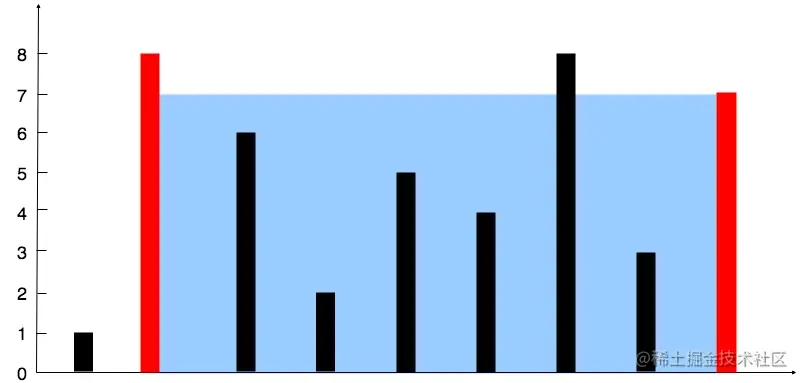``````输入：[1,8,6,2,5,4,8,3,7]

``````

``````输入：height = [1,1]

``````

``````输入：height = [4,3,2,1,4]

``````

• 根据面积计算规则，面积是由两个柱子的距离和柱子最低高度决定的。

• 一开始前后指针指向第一根柱子和最后一根柱子，计算这两根柱子的面积，此时他们距离是最大的。

• 后面的柱子水平距离肯定小于第一根柱子和最后一根柱子的距离，所以只有在高度上，两根柱子更高才有机会比之前的大），再重新计算面积，并和前面的比较，取最大值

``````var maxArea = function(height) {
let left = 0;
let right = height.length - 1;
let result = 0;
while(left < right) {
if(height[left] <= height[right]){
result = Math.max(height[left] * (right - left), result);
left++
} else {
result = Math.max(height[right] * (right - left), result);
right--
}
}
return result;
};
``````

## 三数之和

``````输入：nums = [-1,0,1,2,-1,-4]

``````

``````输入：nums = []

``````

``````输入：nums = 

``````

1. 排序 为什么要排序呢？我想是不是这样：
• 排序后相同的数会挨在一起，对于去除重复的数字有帮助，比如说`[-1,-1,0,2]`其中两个-1和0，2都能3数字之和为0，我们排序之后左边相同的就直接忽略掉了。
1. 遍历
• 使用三个指针 `i、j 和 k` 分别代表要找的三个数。

• 通过枚举 `i` 确定第一个数，另外两个指针 `j，k` 分别从左边 `i + 1` 和右边 `n - 1` 往中间移动，找到满足 `nums[i] + nums[j] + nums[k] == 0` 的所有组合。

• `j``k` 指针的移动逻辑，分情况讨论 `sum = nums[i] + nums[j] + nums[k]`

• sum > 0：k 左移，使 sum 变小

• sum < 0：j 右移，使 sum 变大

• sum = 0：找到符合要求的答案，存起来

``````const threeSum = (nums) => {
nums.sort((a, b) => a-b);
const res = [];
if(nums == null || nums.length< 3){
return [];
}
for(let i = 0; i < nums.length - 2; i++){
const curr = nums[i];
if(curr > 0) break;
if(i - 1 >= 0 && curr === nums[i-1]) continue;
let left = i+1;
let right = nums.length -1;
while(left < right){
let l = nums[left];let r = nums[right];
if(curr + nums[left] + nums[right] === 0){
res.push([curr, nums[left], nums[right]]);
while(left < right && nums[left] === l) left++;
while(left < right && nums[right] === r) right--;
} else if (curr + nums[left] + nums[right] > 0){
right--;
} else {
left++;
}
}
}
return res;
};
``````

## 17 电话号码的字母组合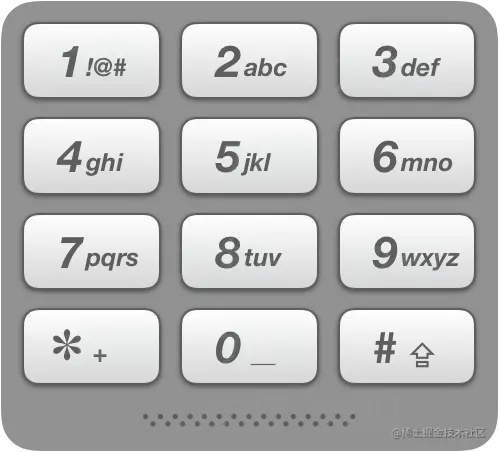``````输入：digits = "23"

``````

``````输入：digits = ""

``````

``````输入：digits = "2"

``````

• 当给定了输入字符串，比如："23"，那么整棵树就构建完成了，如下：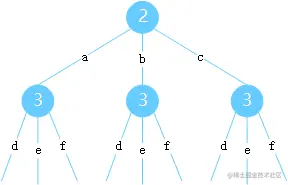``````var letterCombinations = function(digits) {
if (digits.length == 0) return [];
const res = [];
const map = { '2': 'abc', '3': 'def', '4': 'ghi', '5': 'jkl', '6': 'mno', '7': 'pqrs', '8': 'tuv', '9': 'wxyz' };
function dfs(str, deep){
if(str.length === digits.length){
res.push(str);
return;
}
let curr= map[digits[deep]];
for(let i =0; i < curr.length; i++){
dfs(str + curr[i], deep + 1)
}
}
dfs('',0)
return res
};
``````

## 22. 括号生成

``````输入： n = 3

``````

``````输入： n = 1

``````
``````var generateParenthesis = function (n) {
const res = [];

const dfs = (lRemain, rRemain, str) => { // 左右括号所剩的数量，str是当前构建的字符串
if (str.length == 2 * n) { // 字符串构建完成
res.push(str);           // 加入解集
return;                  // 结束当前递归分支
}
if (lRemain > 0) {         // 只要左括号有剩，就可以选它，然后继续做选择（递归）
dfs(lRemain - 1, rRemain, str + "(");
}
if (lRemain < rRemain) {   // 右括号比左括号剩的多，才能选右括号
dfs(lRemain, rRemain - 1, str + ")"); // 然后继续做选择（递归）
}
};

dfs(n, n, ""); // 递归的入口，剩余数量都是n，初始字符串是空串
return res;
};
``````

## 29. 两数相除

``````输入: dividend = 10, divisor = 3

``````

``````输入: dividend = 7, divisor = -3

``````

• 每次以除数作为基数，不断自加，当 sum 逼近到递归的被除数时，记录当前的 count 和剩余的值 (dividend-sum),继续递归

• `divide(10,3) = recur(10,3) = 2 + recur(10-6,3) = 2 + 1 + recur(10-6-3, 3) = 3`

• 注意，递归函数中都是以双整数位基础的，所以最外层调用的时候，要根据入参值进行一定的调整 最后值不能超出 `[-2^31,2^31-1]`

``````var divide = function(dividend, divisor) {
let flag = dividend > 0 && divisor < 0 || dividend < 0 && divisor > 0 ;
dividend = Math.abs(dividend);
divisor = Math.abs(divisor);
function recur(dividend, divisor) {
let count = 1;
let nextDivisor = divisor;
if(dividend < divisor) return 0;
while((nextDivisor + nextDivisor) < dividend){
count += count;
nextDivisor = nextDivisor + nextDivisor;
}
return count + recur(dividend - nextDivisor, divisor);
}
const result =  flag ? -recur(dividend, divisor) : recur(dividend, divisor);
const max = Math.pow(2, 31) - 1, min = -Math.pow(2, 31);
if (result > max) return max
if (result < min) return min
return result;
};
``````

## 字符串转换整数 (atoi)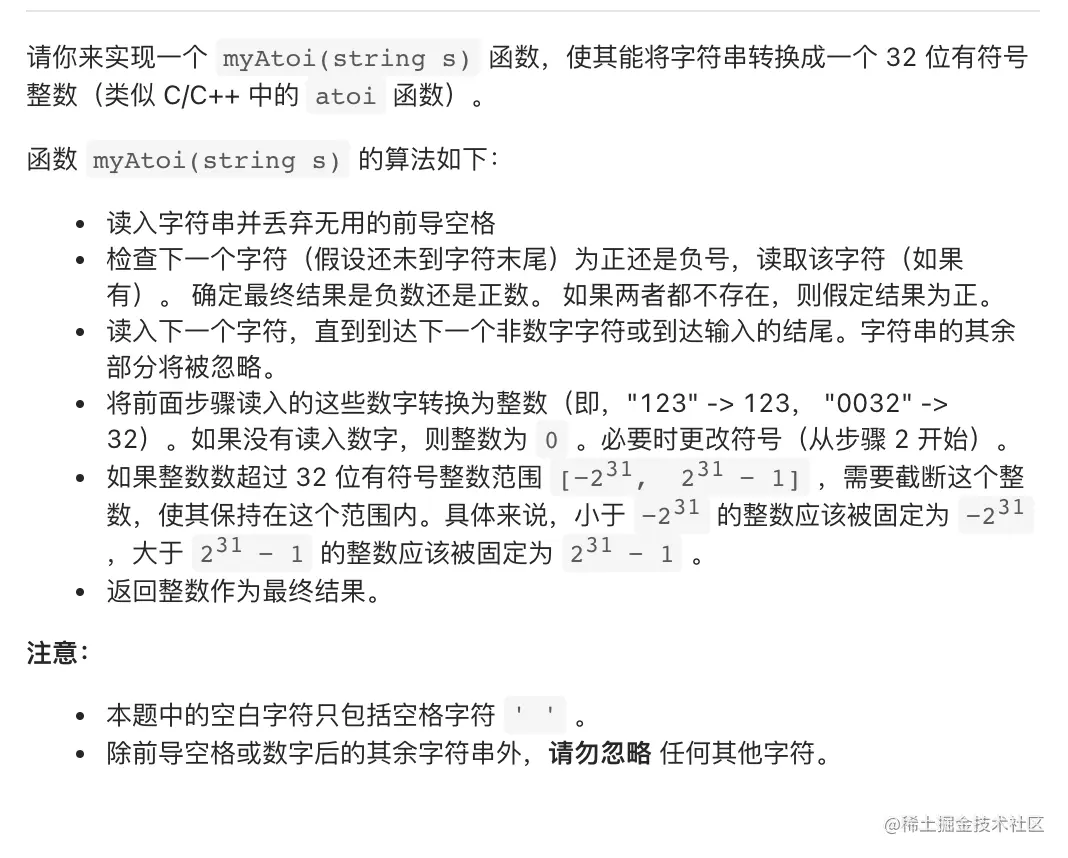``````输入：s = "42"

^

^

^

``````

``````输入：s = "   -42"

^

^

^

``````

``````输入：s = "4193 with words"

^

^

^

``````

``````输入：s = "words and 987"

^

^

^

``````

``````var myAtoi = function(s) {
let result = s.trim().match(/^(\-|\+)?\d+/g);
let res = s.trim().match(/^(\-|\+)?\d+/g);
return res ? Math.max(Math.min(Number(res), 2**31-1), -(2**31)) : 0;
};
``````

## 395. 至少有 K 个重复字符的最长子串

``````输入： s = "aaabb", k = 3

``````

``````输入： s = "ababbc", k = 2

``````
••••••••••